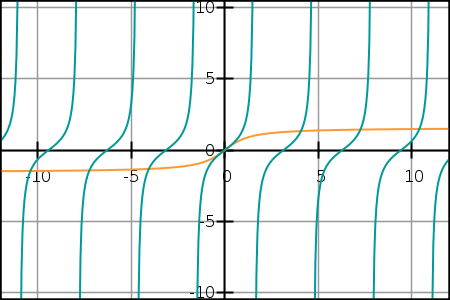Search IntMath
Close

# GraphSketch.com - free online math grapher

By Murray Bourne, 25 Mar 2009

Here's a great service for math students and teachers.

GraphSketch will plot a range of functions including polynomial, trigonometric and exponential ones.

I like the anti-aliased (smooth) edges of the resulting curves — I find it unsatisfactory that most graphers give you chunky, pixelated ones.

Here's an example (it's f(x) = tan x and g(x) = arctan x plotted on the one set of axes.This is a well-executed, free application. The link again: GraphSketch.

[Thanks to JackieB for alerting me to GraphSketch.]

### Comment Preview

HTML: You can use simple tags like <b>, <a href="...">, etc.

To enter math, you can can either:

1. Use simple calculator-like input in the following format (surround your math in backticks, or qq on tablet or phone):
a^2 = sqrt(b^2 + c^2)
(See more on ASCIIMath syntax); or
2. Use simple LaTeX in the following format. Surround your math with $$ and $$.
$$\int g dx = \sqrt{\frac{a}{b}}$$
(This is standard simple LaTeX.)

NOTE: You can mix both types of math entry in your comment.